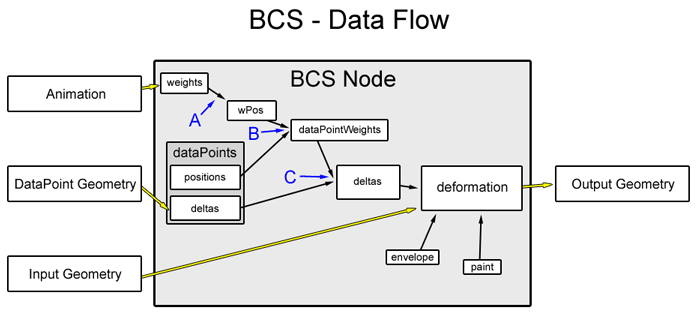# 5.3 Inbetween Basics: WeightPositions

If you take one weight and move it from 0 to 1, you’ll see that the geometry deforms in a linear way, from the neutral shape to the shape defined by the plain dataPoint of the weight. With an inbetween, you can make the object look different at a certain weight value (e.g. 0.5). This can be used to create curved motions, for example for closing the eye or opening the mouth.

In the BCS, the flexibility in how dataPoints are connected to weights makes it possible to achieve this behavior in the same manner that combinations are created. Inbetweens are normal dataPoints that don’t use the position at 1.0 that each weight automatically gets, but a different position, e.g. at 0.5.

To create such an inbetween, you first define a position of the weight by creating a new weightPosition (also wPos or WP) at the value you want the dataPoint to be sitting at. You can even create combination dataPoints at positions containing inbetween WPs.

A weight can have any number of WPs. Each such WP belongs to a certain value of one weight. This value is called its position. If you have one WP at the position 1 of a weight and use it as the position of a (plain) dataPoint, you have created a direct connection between the weight and the dataPoint. This means that the dataPoint behaves like a Blend Shape target.

These WPs create another layer of abstraction, making the diagram of the node still a bit more complicated:You can see that the weights drive the weightPositions (`A`). The values of these weightPositions together with the dataPoint positions are then used to calculate the internal weights of the dataPoints (`B`). At `C`, these dataPointWeights and the dataPoints’ deltas result in the final deltas.